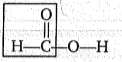# NEET Questions Solved

Which of the following acid gives positive Tollen's reagent test.

(a) $\left({\mathrm{CH}}_{2}{\right)}_{2}{<}_{{\mathrm{CO}}_{2}\mathrm{H}}^{{\mathrm{CO}}_{2}\mathrm{H}}$           (b)  ${\mathrm{CH}}_{2}{<}_{{\mathrm{CO}}_{2}\mathrm{H}}^{{\mathrm{CO}}_{2}\mathrm{H}}$            (c) $\underset{|}{\mathrm{C}}{\mathrm{O}}_{2}\mathrm{H}\phantom{\rule{0ex}{0ex}}{\mathrm{CO}}_{2}\mathrm{H}$              (d) HCO2H

(d)due to formyl like group.

Difficulty Level:

• 15%
• 43%
• 10%
• 34%